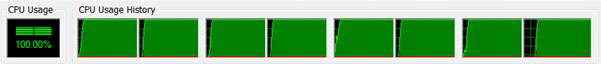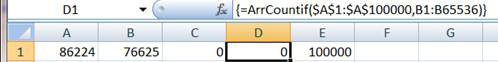You are on the Home/Publications & Training/Case Studies/Array COUNTIF pageShare Your

COUNTIF for a large unsorted array and many searches

Download the add-in There are two add-ins in the zip file, the XLAM for 2007 or later and the XLA for 2003 or earlier.  The VBA project is *not* protected.  To install and load the add-in see Common Installation Instructions.

Ever wanted to count how often each of many numbers (say, 100,000 numbers) occurred in a range of many numbers (say, 100,000)?

Suppose the range to search is A1:A100000 and we want a count of how often each cell in B1:B100000 occurs.  The default approach might be to enter in cell C1 the formula =COUNTIF(\$A:\$A,B1) and copy it down to C2:C100000.  This would give how often each element in B1:B10000 occurs in column A.  And, it will take an awfully long time to calculate – it took about 215 seconds on a multi-threaded 4 processor i7 CPU with Excel using all 8 processors full out.By contrast the ArrCountIf array function below takes about 2 seconds and that too because of a limitation in the Excel VBA interface.  I borrowed the work of others, though both the sort function and the search function are easily written from scratch or found on other sites on the web.

There are a lot of building blocks that were needed to make this work.  In addition to the quick sort and the binary search routines, I also wrote a Transpose routine that both mimics Excel’s TRANSPOSE function and removes the 65,536 row limitation of the native function.  Finally, I added a few array support routines, one to calculate the number of dimensions of an array, and another the length of a given dimension of an array.

A major limitation that arose in developing the code below was that a User Defined Function (UDF) cannot return an array to Excel if the array has more than 65,536 rows.  So, the use of ArrCountIf is limited to ranges of that size.  To use it with 100,000 rows, it has to be entered twice: first, as an array formula in 65,536 cells, and then as another array formula in the remaining 34464 cells.

To use the ArrCountIf function with the model described above, select D1:D65536 and array enter the formula =ArrCountif(\$A\$1:\$A\$100000,B1:B65536).  To array-enter a formula select the range that will contain the formula, enter the formula, and complete it with the CTRL+SHIFT+ENTER combination rather than just the ENTER or TAB key.  If done correctly, Excel will show the formula bracketed in curly brackets { and }.Then, select D65537:D100000 and array enter the formula =ArrCountif(\$A\$1:\$A\$100000,B65537:B100000).

## The Main UDF

In a standard code module enter the code below.

Option Explicit

Option Base 0

Function SearchUpDown(Arr, ByVal Targ, ByVal StartIdx As Long, _

ByVal DirUp As Boolean)

'After a successful binary search, we have the location of 1 _

match.  However, there may be other matches in adjacent array _

locations.  We find them through a linear search

Dim J As Long, Done As Boolean, ThisCount As Long

J = StartIdx

Do

Done = J = IIf(DirUp, UBound(Arr), LBound(Arr))

If Not Done Then

J = J + IIf(DirUp, 1, -1)

If Arr(J) = Targ Then ThisCount = ThisCount + 1 _

Else Done = True

End If

Loop Until Done

SearchUpDown = ThisCount

End Function

Function ArrCOUNTIF(Rng1, Rng2)

'For large searches, ArrCOUNTIF is faster than COUNTIF because it first _

sorts the array being searched.  Consequently, if we want to perform _

the COUNTIF for many values searching a large range, ArrCOUNTIF is _

worth the effort. On the flip side, the overhead of the sort and the _

use of VBA will not be justified for small ranges.

'Returns the result of COUNTIF(Rng2.Cell,Rng1) for each cell in Rng2 _

For some strange reason Rng1 and Rng2 can be ranges as large as the _

1,048,576 rows.  However, when returning the result, we cannot return _

more than 65,536 elements. :(

Dim Arr1, Arr2, Rslt() As Long

'Dim StartTime As Single: StartTime = Timer

Arr1 = Transpose(Rng1.Value)

Arr2 = Transpose(Rng2.Value)

ReDim Rslt(ArrLen(Arr2) - 1)

QuickSort Arr1

Dim I As Long

For I = LBound(Arr2) To UBound(Arr2)

Dim ThisRslt As Long

ThisRslt = BinarySearch(Arr1, Arr2(I))

If ThisRslt >= LBound(Arr1) Then

Rslt(I - LBound(Arr2) + LBound(Rslt)) = _

1 + SearchUpDown(Arr1, Arr2(I), ThisRslt, DirUp:=False) _

+ SearchUpDown(Arr1, Arr2(I), ThisRslt, DirUp:=True)

End If

Next I

ArrCOUNTIF = Transpose(Rslt)

'Debug.Print ArrLen(Arr1); ArrLen(Arr2); Timer - StartTime

End Function

## The Quick Sort support module

In a standard module, enter the code below.  It performs the quick sort needed to sort the range being searched.

Option Explicit

Private Sub noCheckQuickSort(ByRef SortArray, L, R)

'Originally posted to an XL NG by Jim Rech

Dim I, J, x, y

I = L

J = R

x = SortArray((L + R) / 2)

While (I <= J)

While (SortArray(I) < x And I < R)

I = I + 1

Wend

While (x < SortArray(J) And J > L)

J = J - 1

Wend

If (I <= J) Then

y = SortArray(I)

SortArray(I) = SortArray(J)

SortArray(J) = y

I = I + 1

J = J - 1

End If

Wend

If (L < J) Then Call noCheckQuickSort(SortArray, L, J)

If (I < R) Then Call noCheckQuickSort(SortArray, I, R)

End Sub

Public Sub QuickSort(ByRef Arr, Optional L, Optional R)

If InStr(1, TypeName(Arr), "(", vbTextCompare) < 1 Then Exit Sub

If IsMissing(L) Then L = LBound(Arr)

If IsMissing(R) Then R = UBound(Arr)

If Not IsNumeric(L) Then Exit Sub

If Not IsNumeric(R) Then Exit Sub

If Int(L) <> L Then Exit Sub

If Int(R) <> R Then Exit Sub

If L >= R Then Exit Sub

If L < LBound(Arr) Then Exit Sub

If R > UBound(Arr) Then Exit Sub

noCheckQuickSort Arr, L, R

End Sub

## The Transpose support module

In a standard code module enter the code below.  It mimics Excel’s TRANSPOSE function in VBA overcoming the limitation that the built-in function is limited to 65,536 rows.

Option Explicit

Function OneDto2D(Arr)

Dim I As Long, Rslt()

ReDim Rslt(ArrLen(Arr) - 1, 0)

For I = LBound(Arr) To UBound(Arr)

Rslt(I - LBound(Arr) + LBound(Rslt), 0) = Arr(I)

Next I

OneDto2D = Rslt

End Function

Function TwoDto1D(Arr)

Dim I As Long, Rslt()

ReDim Rslt(ArrLen(Arr) - 1)

For I = LBound(Arr) To UBound(Arr)

Rslt(I - LBound(Arr) + LBound(Rslt)) = Arr(I, LBound(Arr, 2))

Next I

TwoDto1D = Rslt

End Function

Dim I As Long, J As Long, Rslt()

ReDim Rslt(ArrLen(Arr, 2) - 1, ArrLen(Arr, 1) - 1)

For I = LBound(Arr) To UBound(Arr)

For J = LBound(Arr, 2) To UBound(Arr, 2)

Rslt(J - LBound(Arr, 2) + LBound(Rslt, 1), _

I - LBound(Arr, 1) + LBound(Rslt, 2)) = Arr(I, J)

Next J

Next I

End Function

Function Transpose(Arr)

'This mimics Excel's the effect of _

Application.WorksheetFunction.Transpose except that it works with _

more than 65,536 rows.  The Excel Transposefunction does something _

strange in that it transforms a 2D N-rows-1-column matrix into a _

1D array of N elements.  In reverse, it transforms a 1D array of N _

elements into a 2D N-rows-1-column matrix.

Select Case NbrDim(Arr)

Case 1: Transpose = OneDto2D(Arr)

Case 2:

If ArrLen(Arr, 2) = 1 Then Transpose = TwoDto1D(Arr) _

Case Is > 2:

'The function is not defined for matrices with >2 dimensions

Case Else:

'The function is not defined for non-matrices

End Select

End Function

## The Binary Search and Array Support module

In a standard code module enter the code below.  It implements the binary search function and includes functions for working with arrays.

Option Explicit

Function ArrLen(Arr, Optional ByVal aDim As Integer = 1)

End Function

Function NbrDim(Arr)

NbrDim = -1

On Error GoTo XIT

Dim I: I = 1

Do While True

Dim aLen: aLen = UBound(Arr, I)

I = I + 1

Loop

XIT:

NbrDim = I - 1

End Function

Function BinarySearch(Arr As Variant, Targ As Variant, _

Optional ByVal inLow, Optional ByVal inHigh) As Long

'Binary search code is easily found through a web search. _

This implementation is based on http://www.devx.com/vb2themax/Tip/18913

Dim Low As Long, High As Long

If IsMissing(inLow) Then Low = LBound(Arr) Else Low = inLow

If IsMissing(inHigh) Then High = UBound(Arr) Else High = inHigh

BinarySearch = LBound(Arr) - 1

If Low > High Or Low < LBound(Arr) Or High > UBound(Arr) Then _

Exit Function

Dim DescOrder As Boolean: DescOrder = (Arr(Low) > Arr(High))

'A good faith guess

Do

Dim Mid As Long

Mid = (Low + High) \ 2

If Arr(Mid) = Targ Then

BinarySearch = Mid

Exit Do

ElseIf ((Arr(Mid) < Targ) Xor DescOrder) Then

Low = Mid + 1

Else

High = Mid - 1

End If

Loop Until Low > High

End Function